# R S Aggarwal Solutions for Class 10 Maths Chapter 14 Height and Distance

R S Aggarwal Class 10 Solutions for Chapter 14 Height and Distance are available here. This set of important questions helps students to master height and distance problems. All solutions have been designed considering the concept-based approach, which allows students to answer precisely any of the questions. Diagrams are provided wherever necessary to promote visual learning and better understanding. To score high marks students are suggested to download R S Aggarwal Class 10 maths and solve all the questions.

## Download PDF of R S Aggarwal Solutions for Class 10 Chapter 14 Height and Distance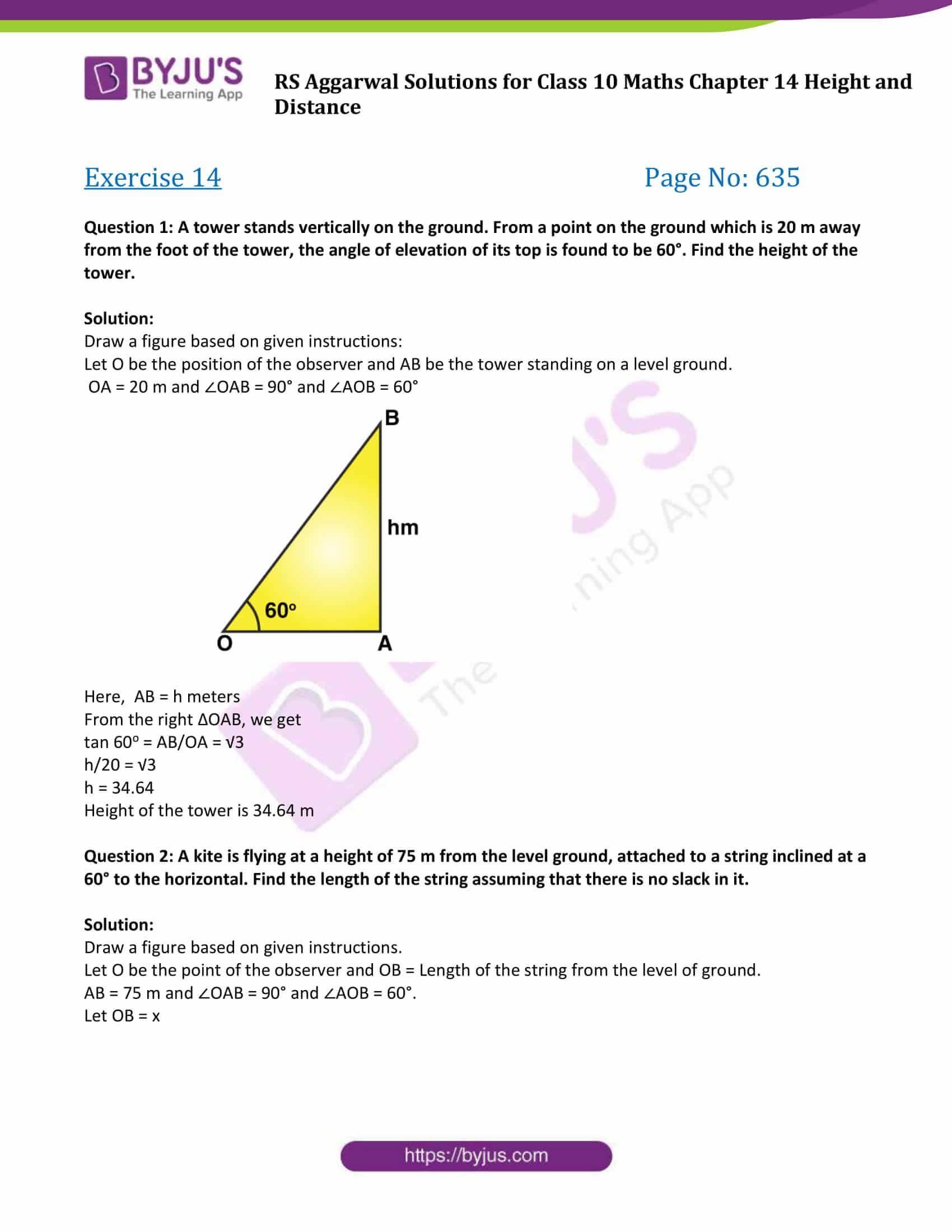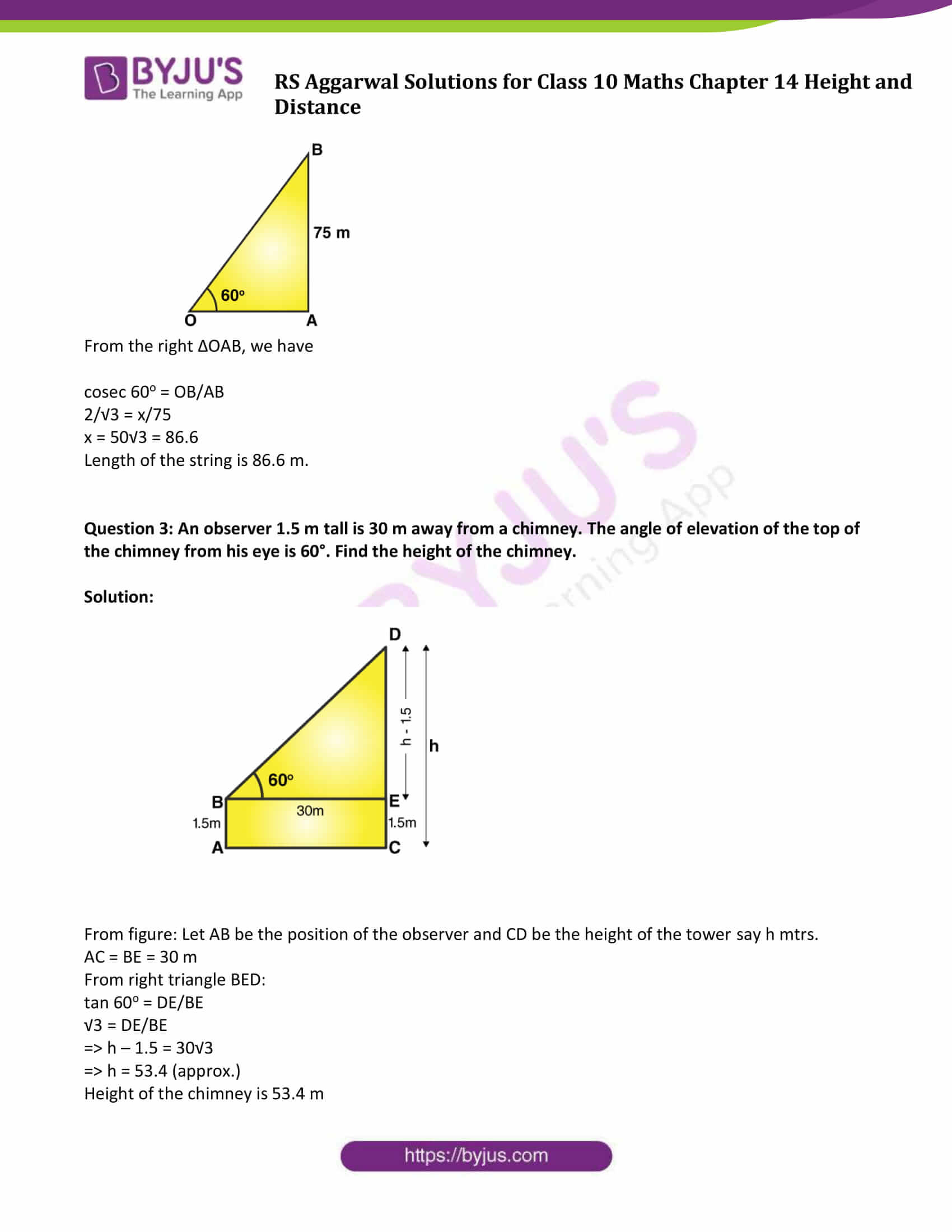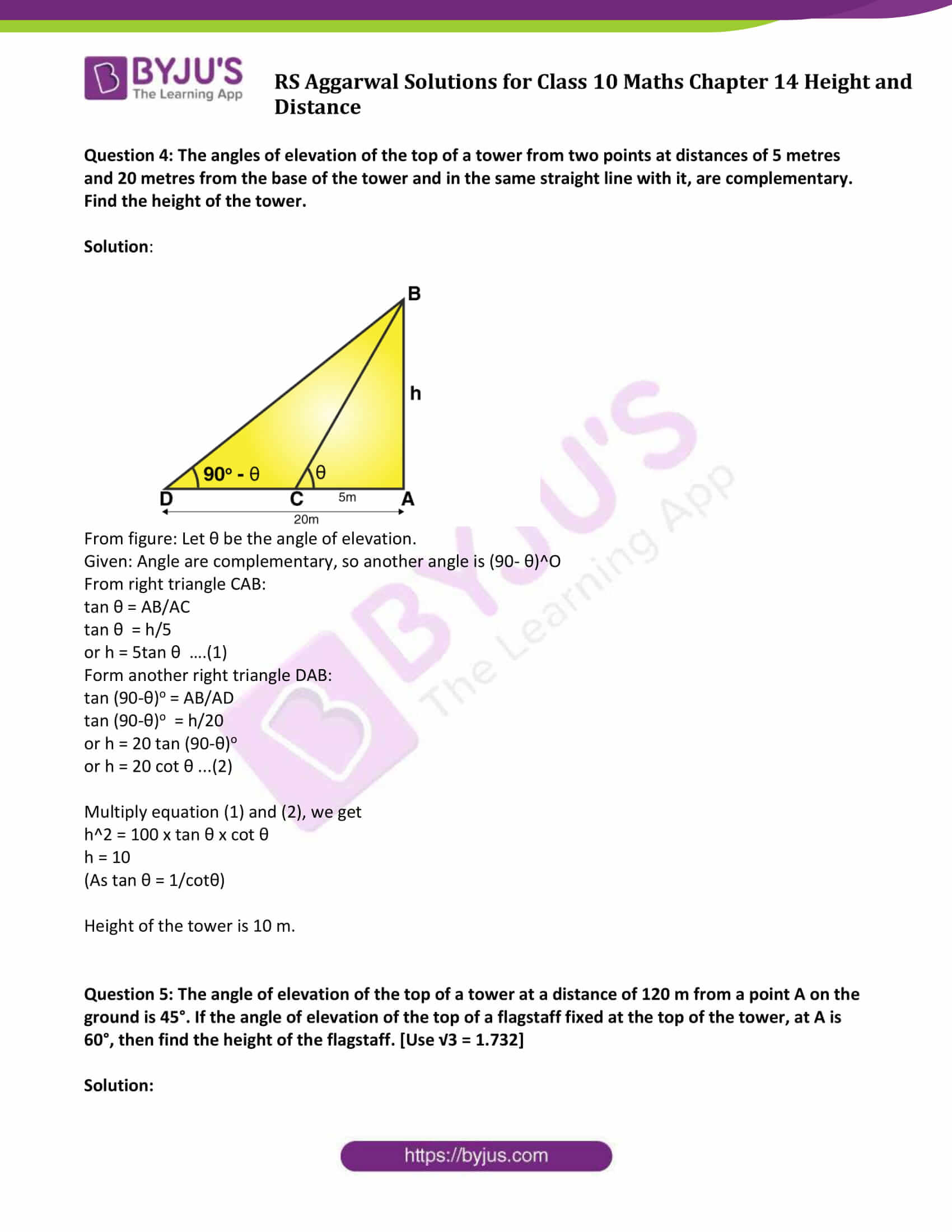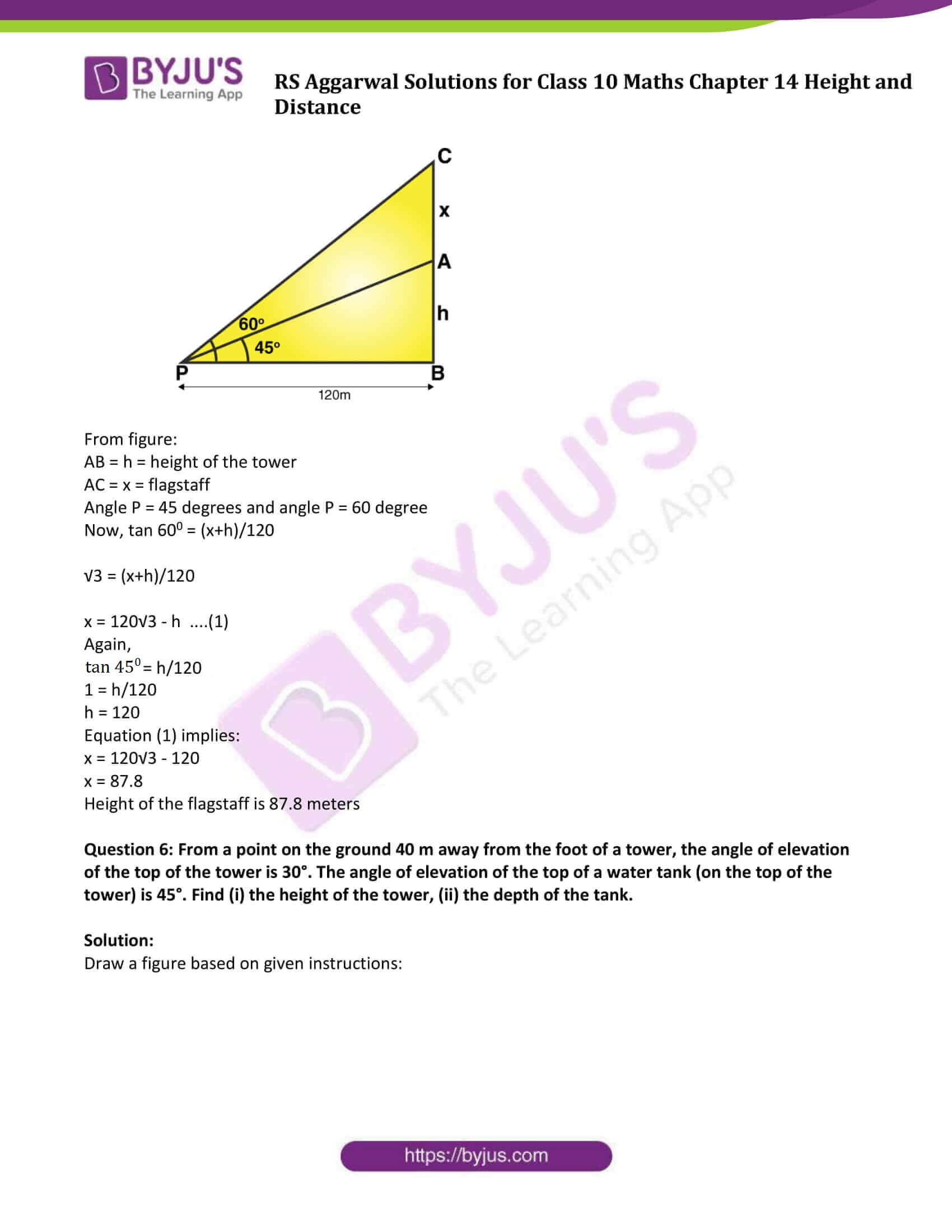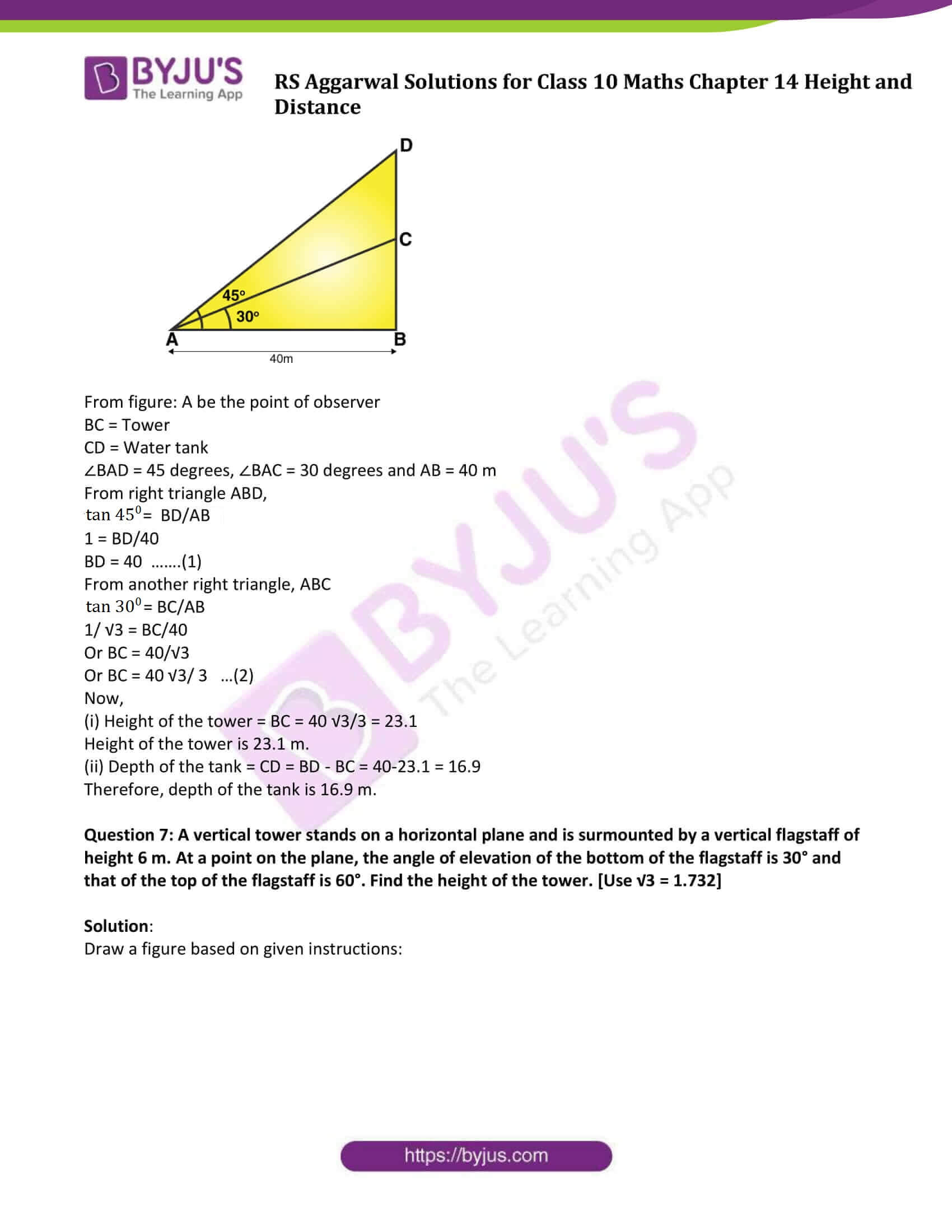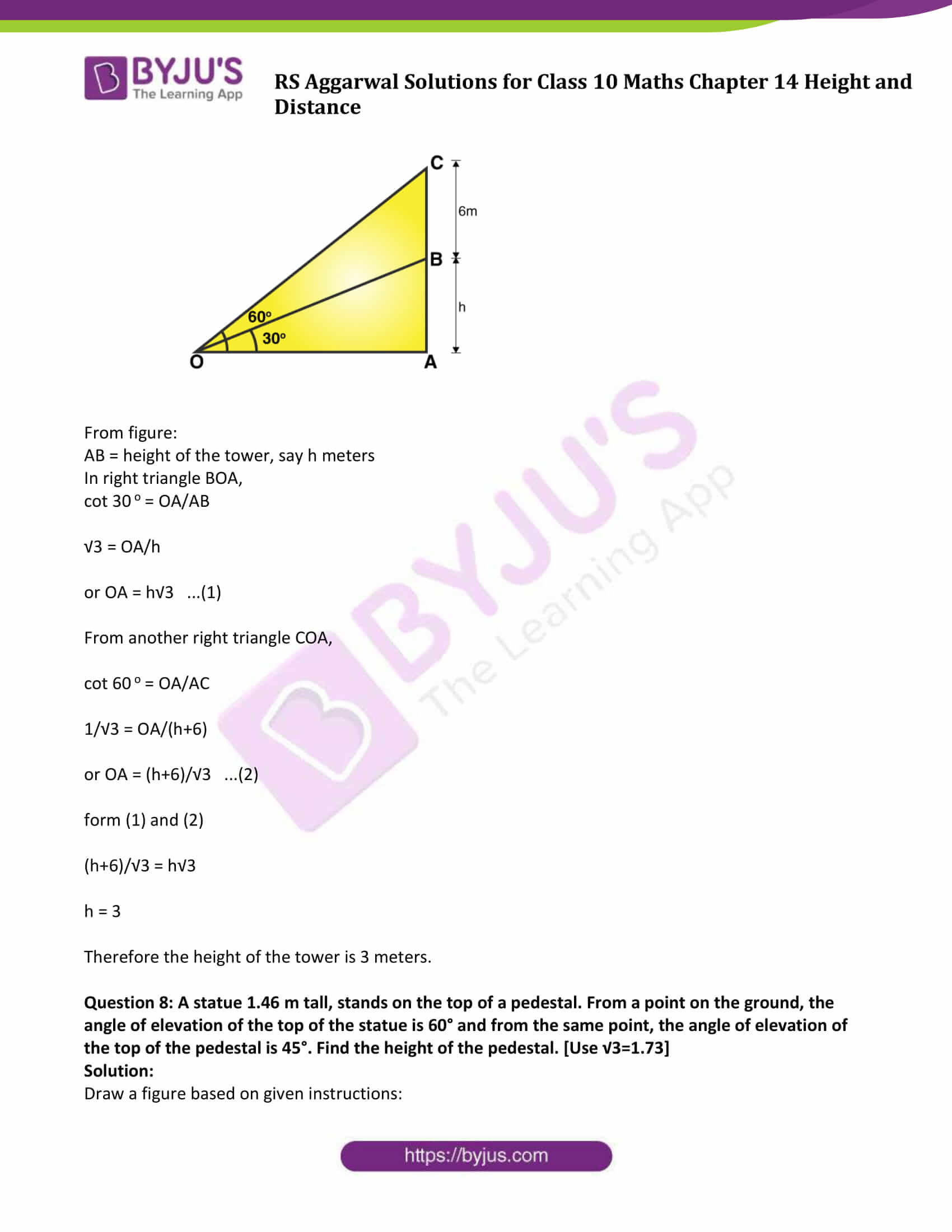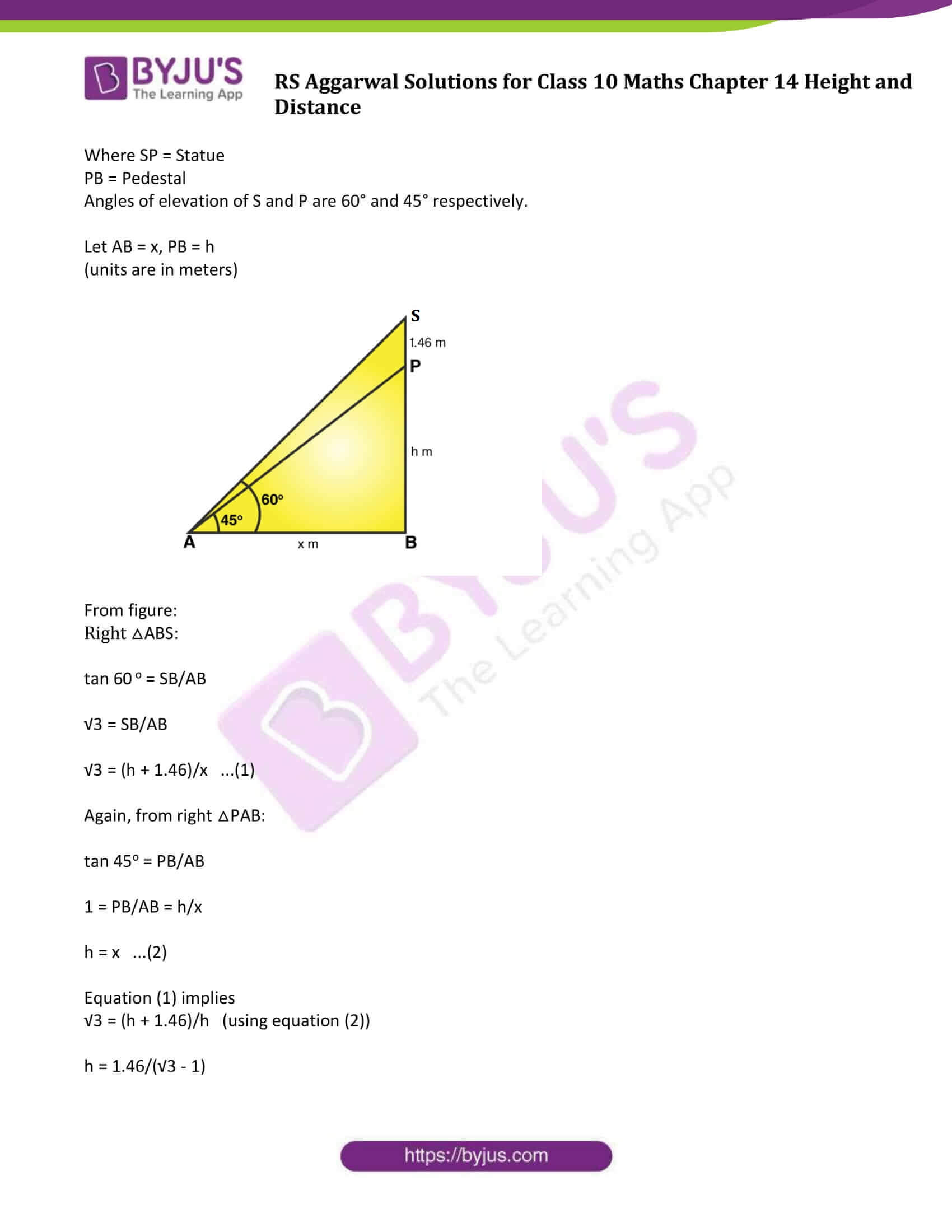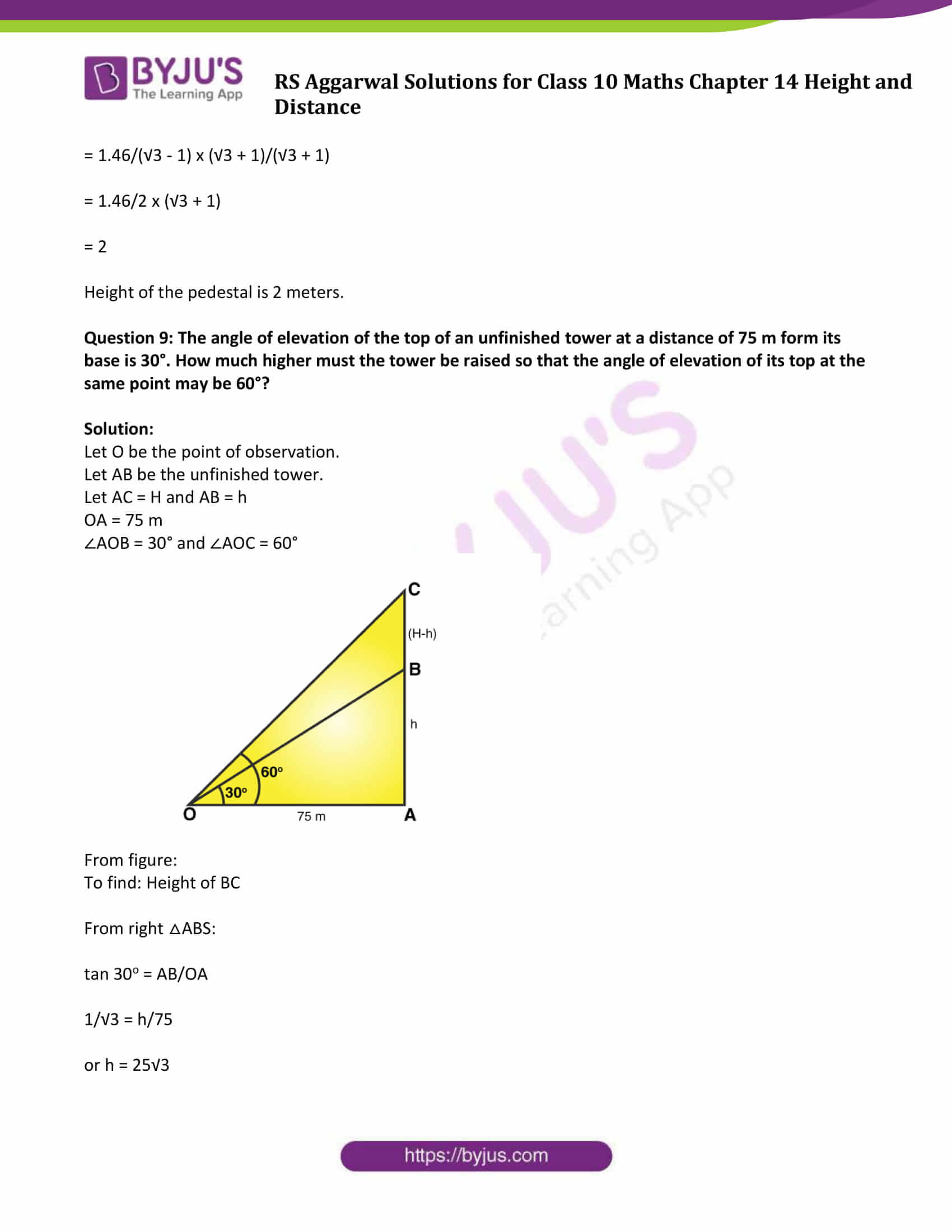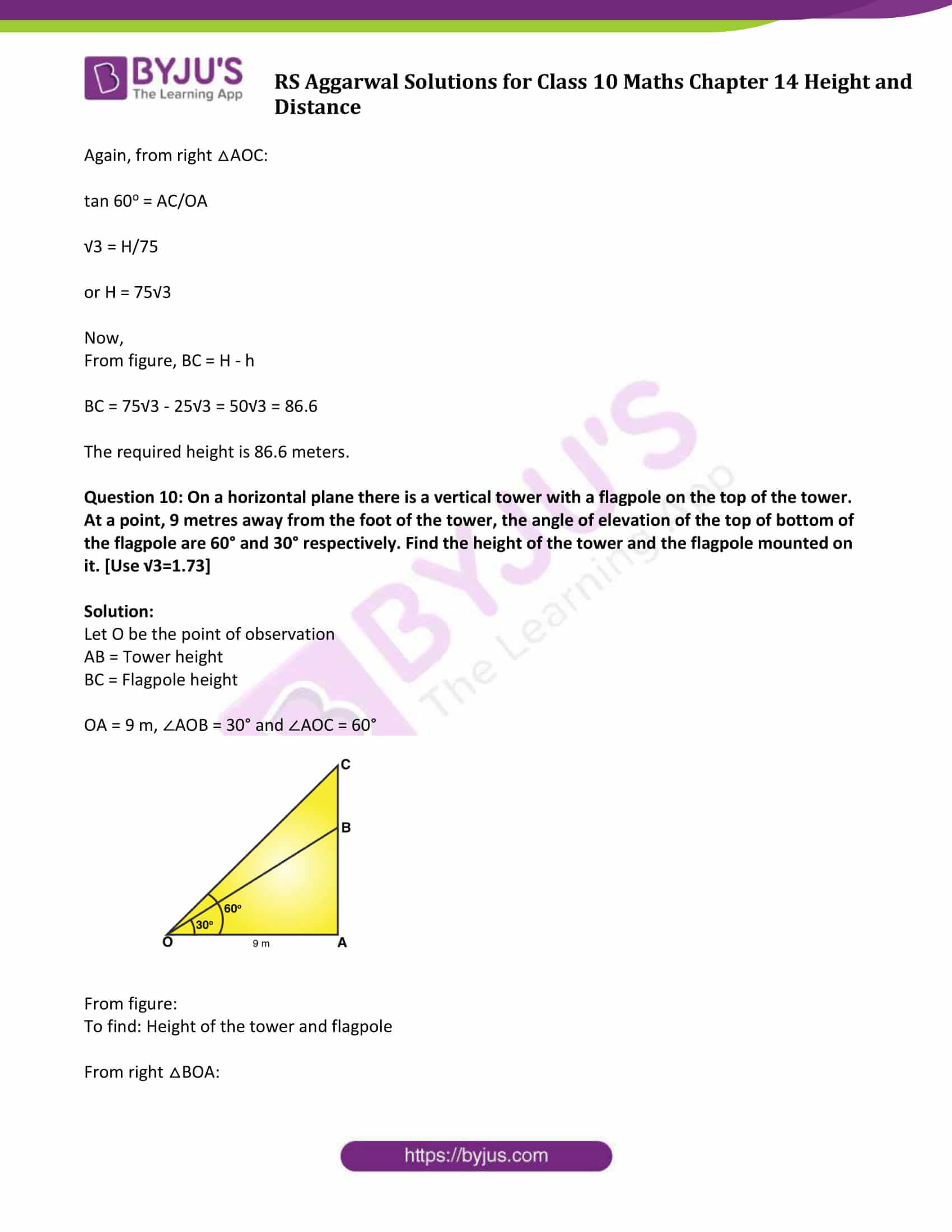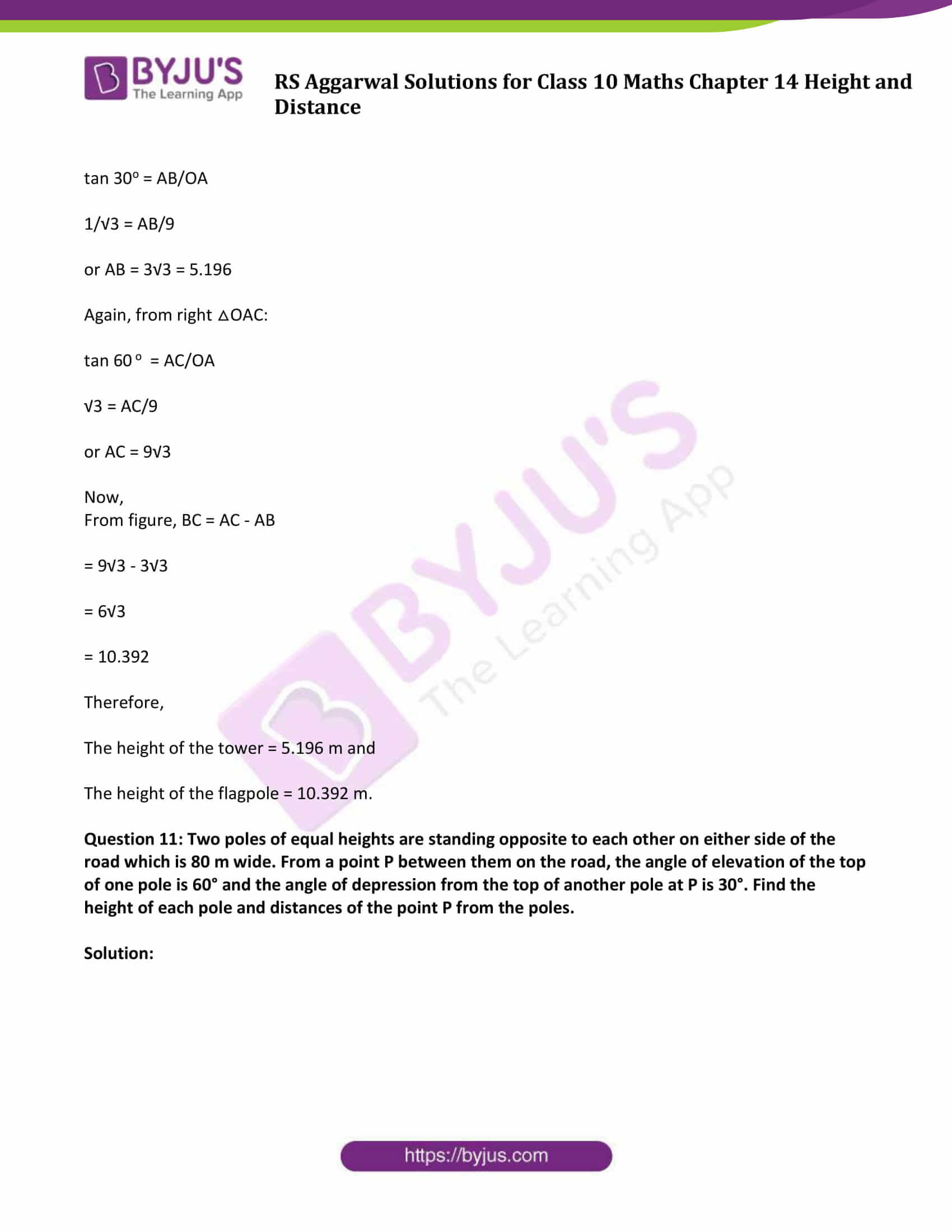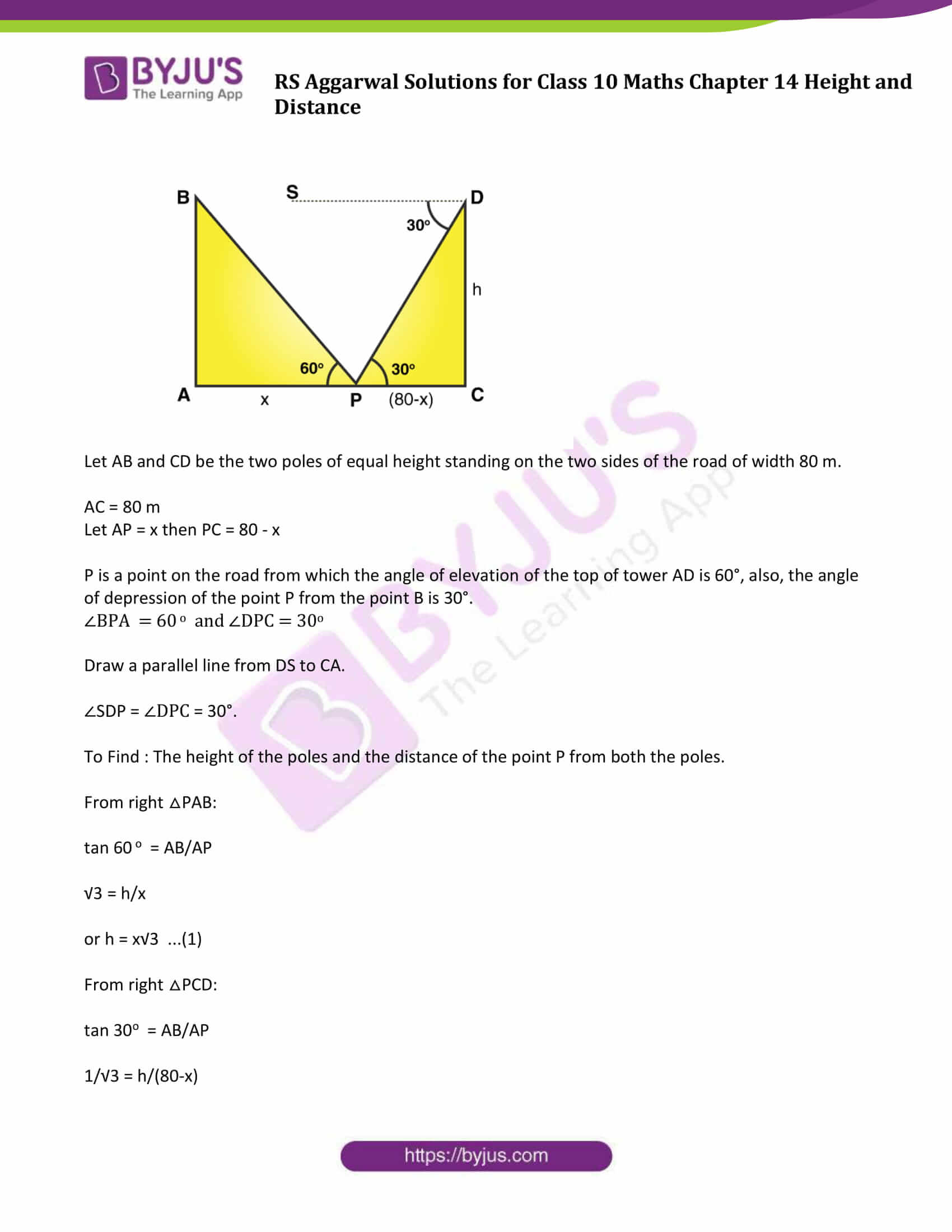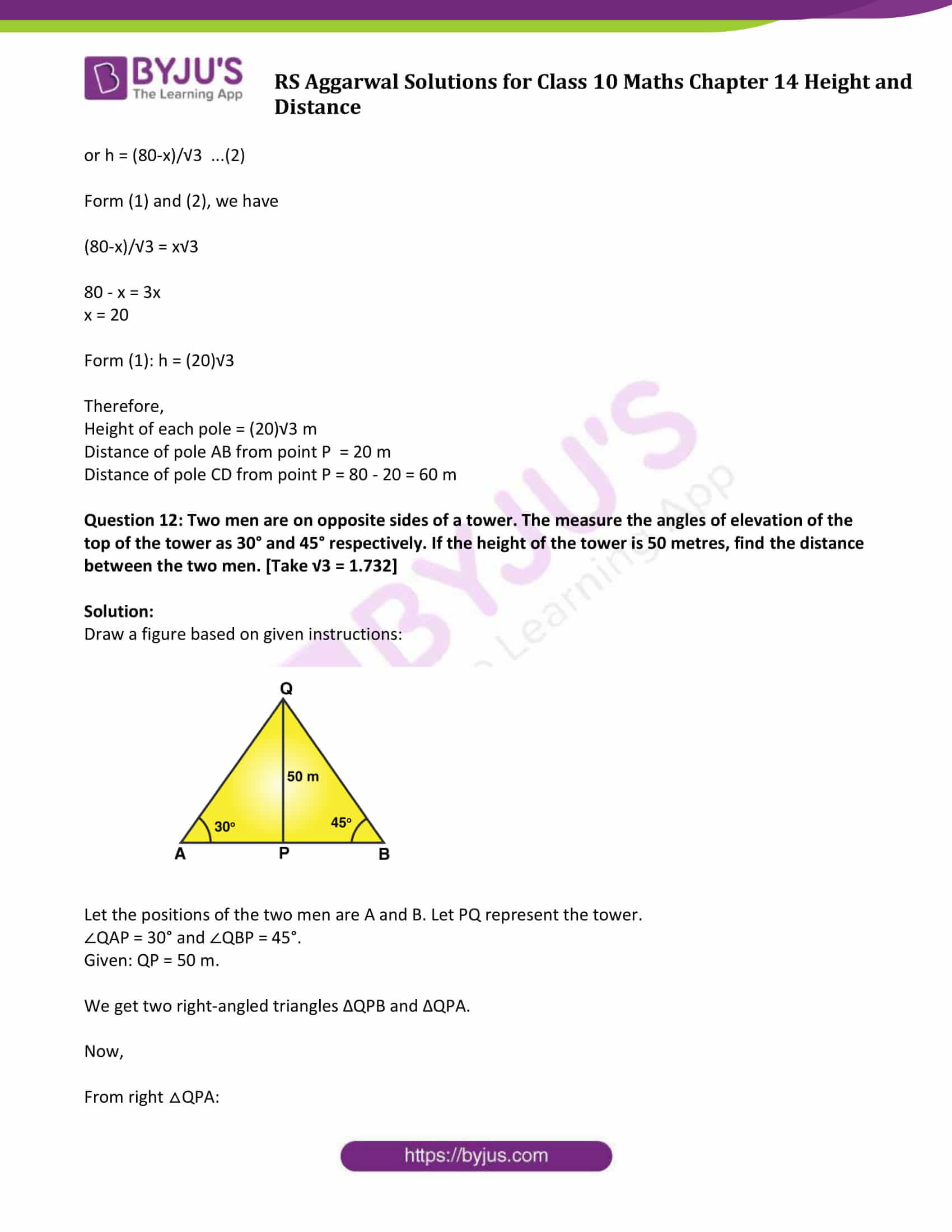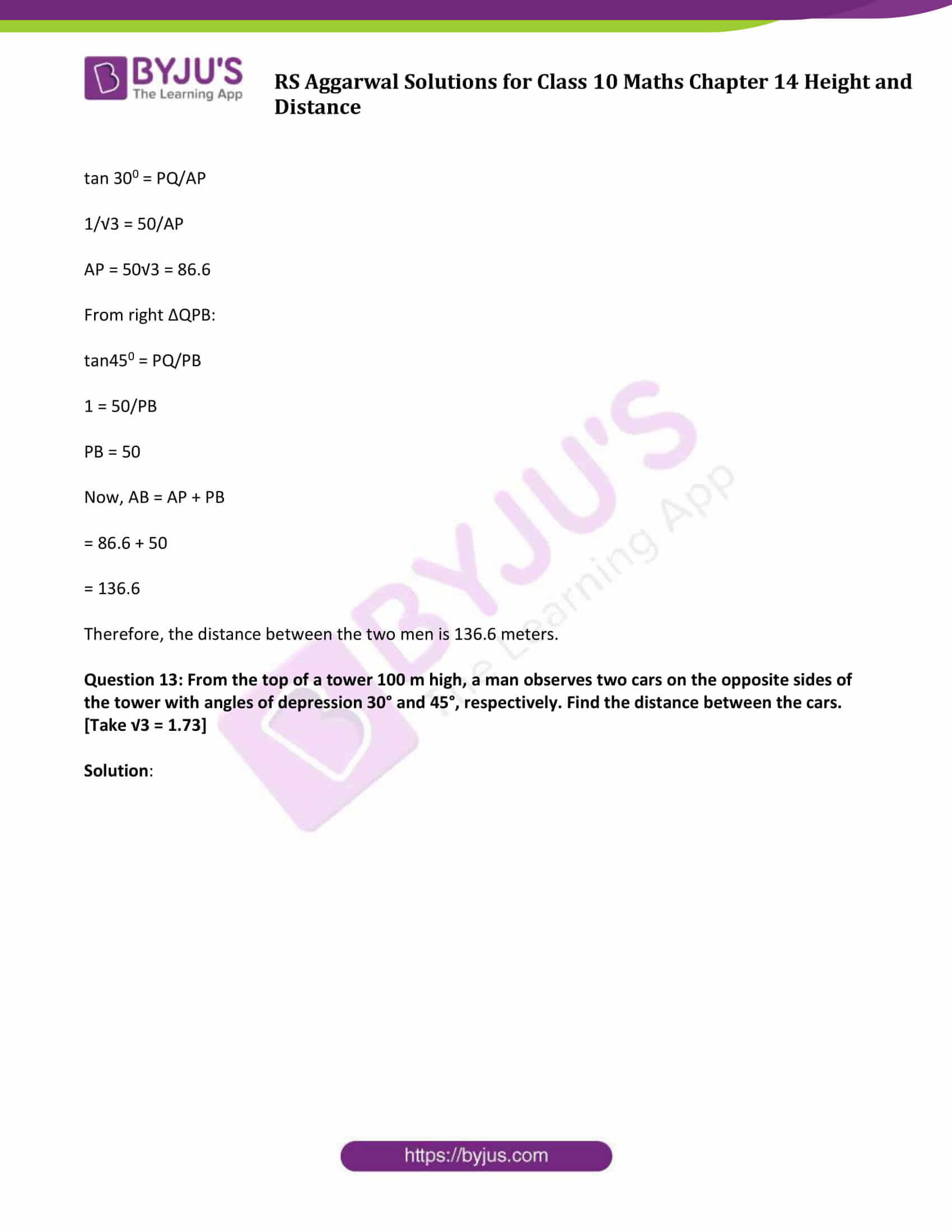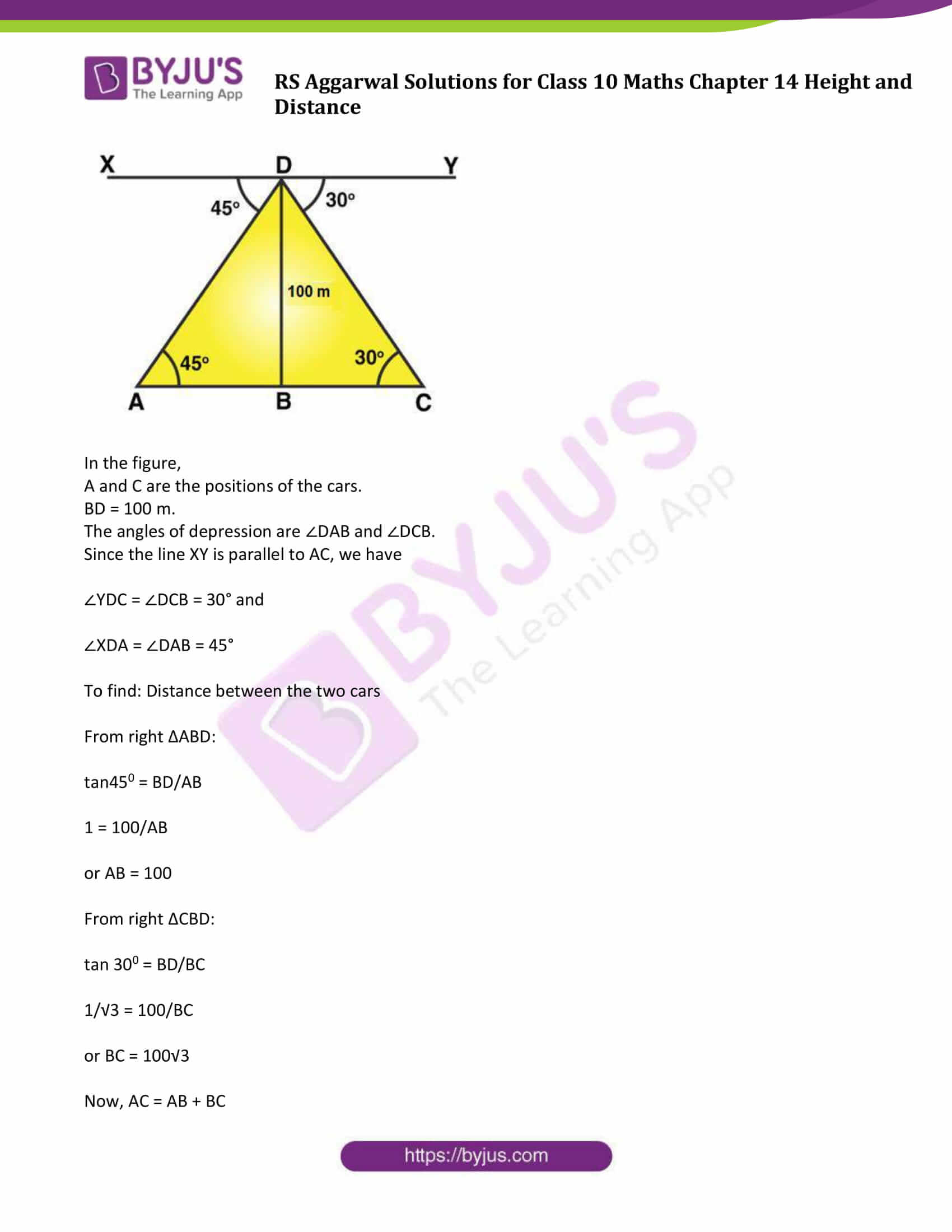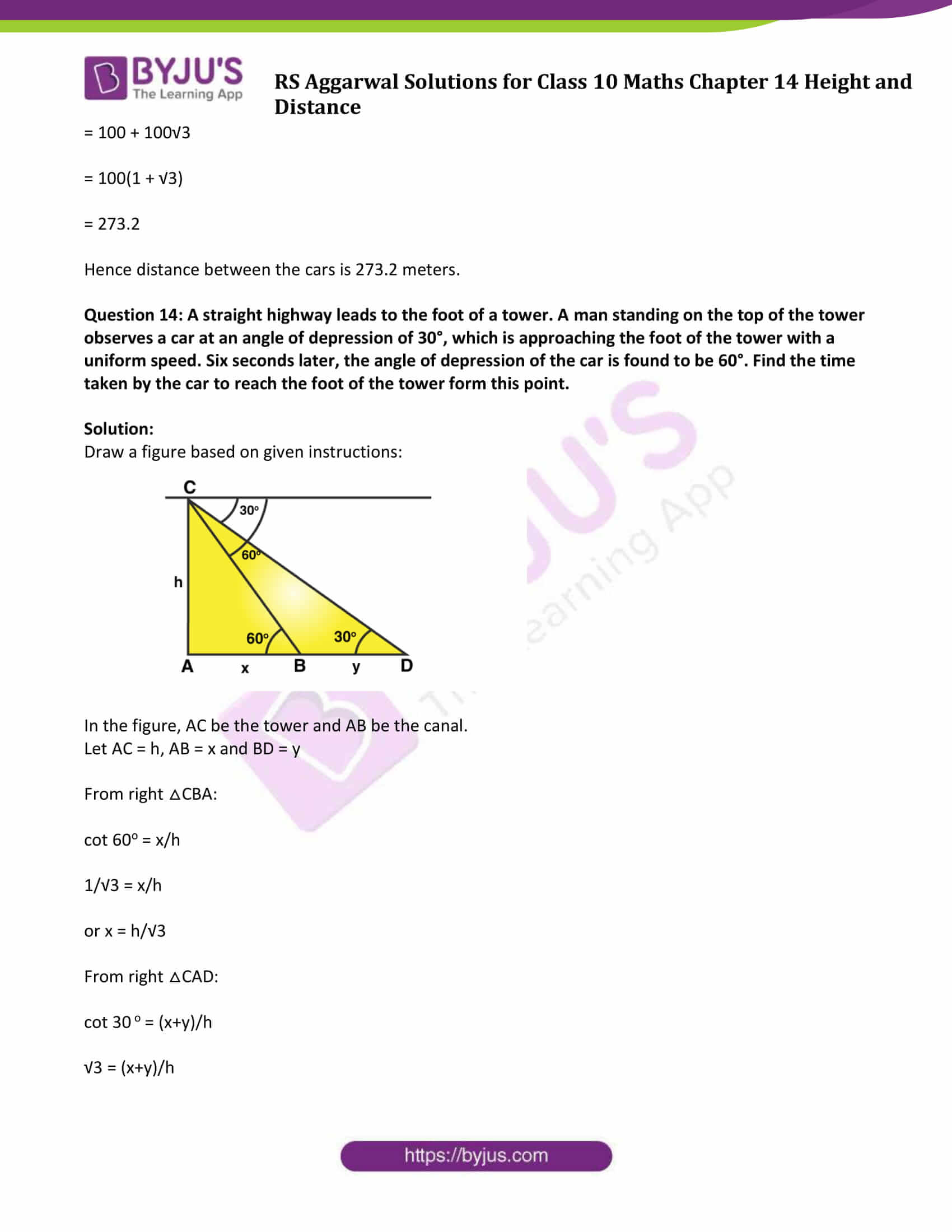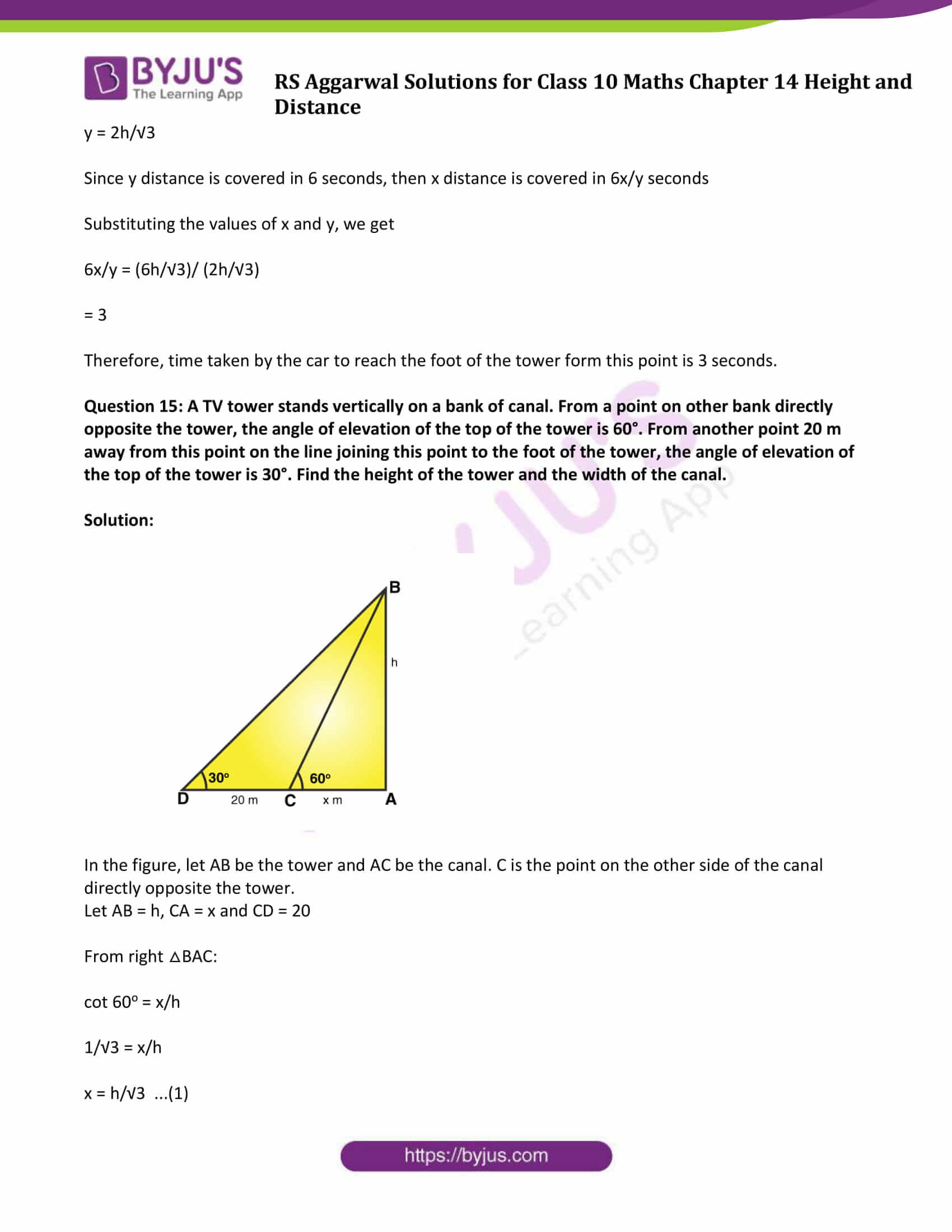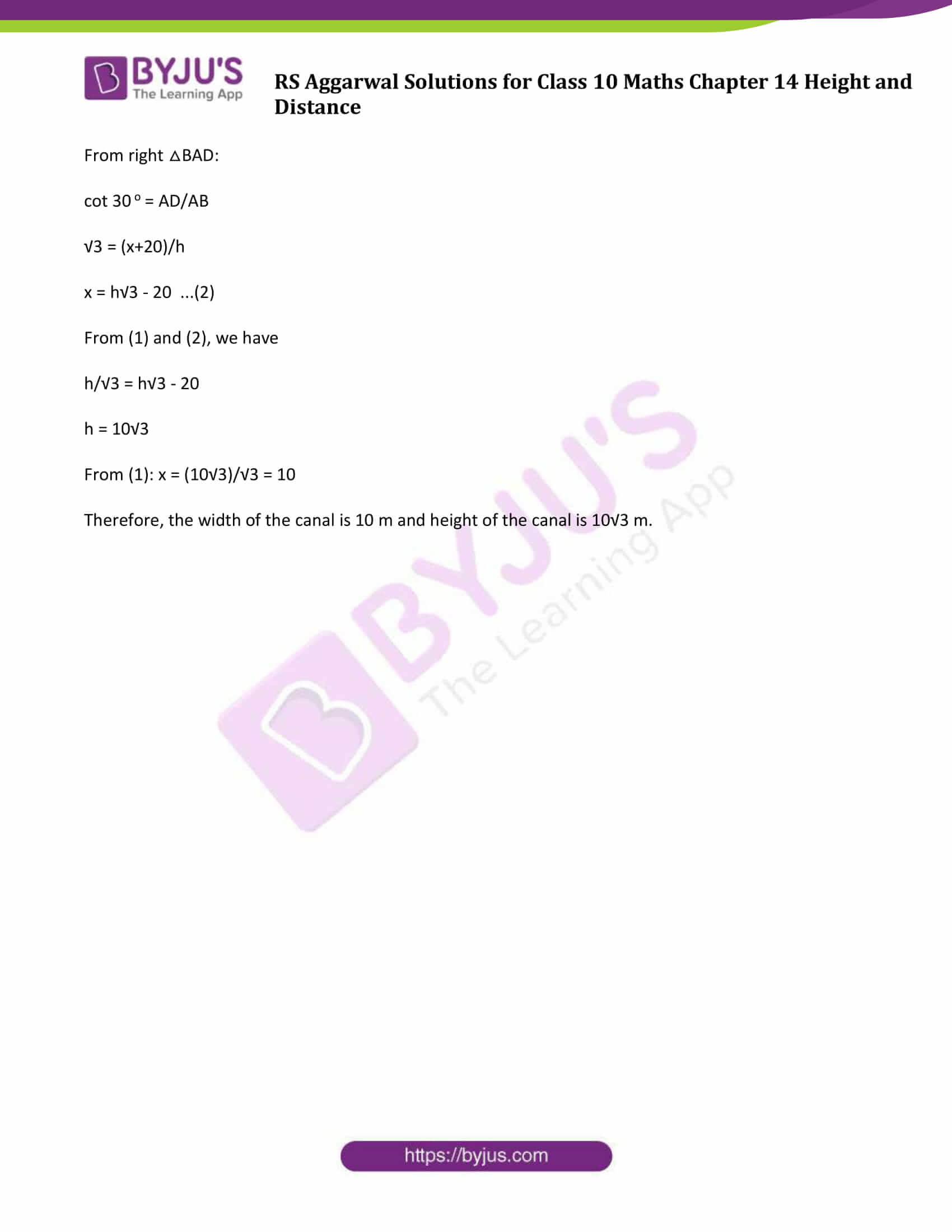### Access Answers to Maths R S Aggarwal Chapter 14 – Height and Distance

Question 1: A tower stands vertically on the ground. From a point on the ground which is 20 m away from the foot of the tower, the angle of elevation of its top is found to be 60°. Find the height of the tower.

Solution:

Draw a figure based on given instructions:

Let O be the position of the observer and AB be the tower standing on a level ground.

OA = 20 m and ∠OAB = 90° and ∠AOB = 60°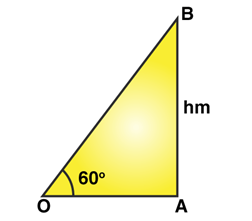Here, AB = h meters

From the right ∆OAB, we get

tan 60o = AB/OA = √3

h/20 = √3

h = 34.64

Height of the tower is 34.64 m

Question 2: A kite is flying at a height of 75 m from the level ground, attached to a string inclined at a 60° to the horizontal. Find the length of the string assuming that there is no slack in it.

Solution:

Draw a figure based on given instructions.

Let O be the point of the observer and OB = Length of the string from the level of ground.

AB = 75 m and ∠OAB = 90° and ∠AOB = 60°.

Let OB = x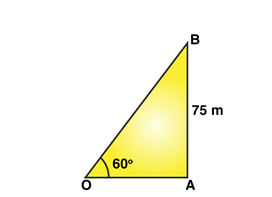From the right ∆OAB, we have

cosec 60o = OB/AB

2/√3 = x/75

x = 50√3 = 86.6

Length of the string is 86.6 m.

Question 3: An observer 1.5 m tall is 30 m away from a chimney. The angle of elevation of the top of the chimney from his eye is 60°. Find the height of the chimney.

Solution: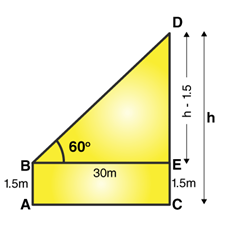From figure: Let AB be the position of the observer and CD be the height of the tower say h mtrs.

AC = BE = 30 m

From right triangle BED:

tan 60o = DE/BE

√3 = DE/BE

=> h – 1.5 = 30√3

=> h = 53.4 (approx.)

Height of the chimney is 53.4 m

Question 4: The angles of elevation of the top of a tower from two points at distances of 5 metres and 20 metres from the base of the tower and in the same straight line with it, are complementary. Find the height of the tower.

Solution: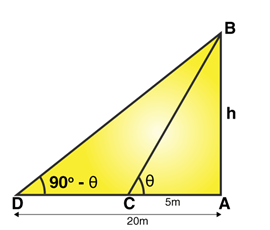From figure: Let θ be the angle of elevation.

Given: Angle are complementary, so another angle is (90- θ)^O

From right triangle CAB:

tan θ = AB/AC

tan θ = h/5

or h = 5tan θ ….(1)

Form another right triangle DAB:

tan (90-θ)o = h/20

or h = 20 tan (90-θ)o

or h = 20 cot θ …(2)

Multiply equation (1) and (2), we get

h^2 = 100 x tan θ x cot θ

h = 10

(As tan θ = 1/cotθ)

Height of the tower is 10 m.

Question 5: The angle of elevation of the top of a tower at a distance of 120 m from a point A on the ground is 45°. If the angle of elevation of the top of a flagstaff fixed at the top of the tower, at A is 60°, then find the height of the flagstaff. [Use √3 = 1.732]

Solution: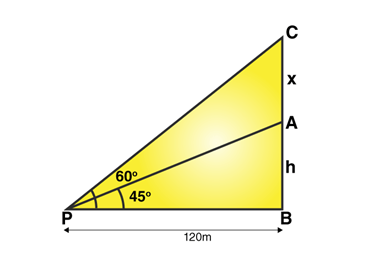From figure:

AB = h = height of the tower

AC = x = flagstaff

Angle P = 45 degrees and angle P = 60 degree

Now, tan 600 = (x+h)/120

√3 = (x+h)/120

x = 120√3 – h ….(1)

Again,

tan 45o = h/120

1 = h/120

h = 120

Equation (1) implies:

x = 120√3 – 120

x = 87.8

Height of the flagstaff is 87.8 meters

Question 6: From a point on the ground 40 m away from the foot of a tower, the angle of elevation of the top of the tower is 30°. The angle of elevation of the top of a water tank (on the top of the tower) is 45°. Find (i) the height of the tower, (ii) the depth of the tank.

Solution:

Draw a figure based on given instructions: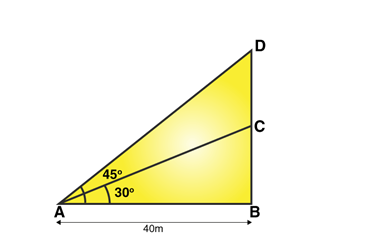From figure: A be the point of observer

BC = Tower

CD = Water tank

∠BAD = 45 degrees, ∠BAC = 30 degrees and AB = 40 m

From right triangle ABD,

tan 45o = BD/AB

1 = BD/40

BD = 40 …….(1)

From another right triangle, ABC

Tan 30o = BC/AB

1/ √3 = BC/40

Or BC = 40/√3

Or BC = 40 √3/ 3 …(2)

Now,

(i) Height of the tower = BC = 40 √3/3 = 23.1

Height of the tower is 23.1 m.

(ii) Depth of the tank = CD = BD – BC = 40-23.1 = 16.9

Therefore, depth of the tank is 16.9 m.

Question 7: A vertical tower stands on a horizontal plane and is surmounted by a vertical flagstaff of height 6 m. At a point on the plane, the angle of elevation of the bottom of the flagstaff is 30° and that of the top of the flagstaff is 60°. Find the height of the tower. [Use √3 = 1.732]

Solution:

Draw a figure based on given instructions: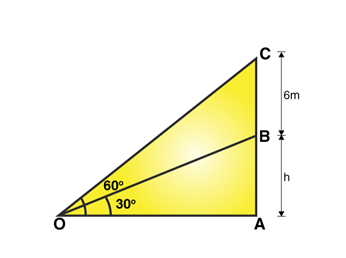From figure:

AB = height of the tower, say h meters

In right triangle BOA,

cot 30 o = OA/AB

√3 = OA/h

or OA = h√3 …(1)

From another right triangle COA,

cot 60 o = OA/AC

1/√3 = OA/(h+6)

or OA = (h+6)/√3 …(2)

form (1) and (2)

(h+6)/√3 = h√3

h = 3

Therefore the height of the tower is 3 meters.

Question 8: A statue 1.46 m tall, stands on the top of a pedestal. From a point on the ground, the angle of elevation of the top of the statue is 60° and from the same point, the angle of elevation of the top of the pedestal is 45°. Find the height of the pedestal. [Use √3=1.73]

Solution:

Draw a figure based on given instructions:

Where SP = Statue

PB = Pedestal

Angles of elevation of S and P are 60° and 45° respectively.

Let AB = x, PB = h

(units are in meters)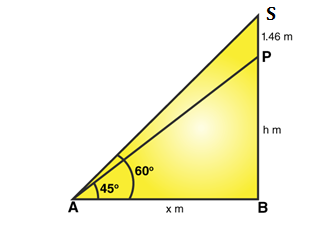From figure:

Right △ABS:

tan 60 o = SB/AB

√3 = SB/AB

√3 = (h + 1.46)/x …(1)

Again, from right △PAB:

tan 45o = PB/AB

1 = PB/AB = h/x

h = x …(2)

Equation (1) implies

√3 = (h + 1.46)/h (using equation (2))

h = 1.46/(√3 – 1)

= 1.46/(√3 – 1) x (√3 + 1)/(√3 + 1)

= 1.46/2 x (√3 + 1)

= 2

Height of the pedestal is 2 meters.

Question 9: The angle of elevation of the top of an unfinished tower at a distance of 75 m form its base is 30°. How much higher must the tower be raised so that the angle of elevation of its top at the same point may be 60°?

Solution:

Let O be the point of observation.

Let AB be the unfinished tower.

Let AC = H and AB = h

OA = 75 m

∠AOB = 30° and ∠AOC = 60°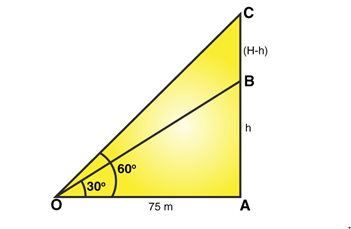From figure:

To find: Height of BC

From right △ABS:

tan 30o = AB/OA

1/√3 = h/75

or h = 25√3

Again, from right △AOC:

tan 60o = AC/OA

√3 = H/75

or H = 75√3

Now,

From figure, BC = H – h

BC = 75√3 – 25√3 = 50√3 = 86.6

The required height is 86.6 meters.

Question 10: On a horizontal plane there is a vertical tower with a flagpole on the top of the tower. At a point, 9 metres away from the foot of the tower, the angle of elevation of the top of bottom of the flagpole are 60° and 30° respectively. Find the height of the tower and the flagpole mounted on it. [Use √3=1.73]

Solution:

Let O be the point of observation

AB = Tower height

BC = Flagpole height

OA = 9 m, ∠AOB = 30° and ∠AOC = 60°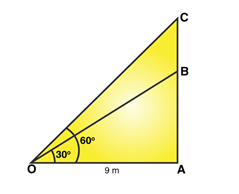From figure:

To find: Height of the tower and flagpole

From right △BOA:

tan 30o = AB/OA

1/√3 = AB/9

or AB = 3√3 = 5.196

Again, from right △OAC:

tan 60 o = AC/OA

√3 = AC/9

or AC = 9√3

Now,

From figure, BC = AC – AB

= 9√3 – 3√3

= 6√3

= 10.392

Therefore,

The height of the tower = 5.196 m and

The height of the flagpole = 10.392 m.

Question 11: Two poles of equal heights are standing opposite to each other on either side of the road which is 80 m wide. From a point P between them on the road, the angle of elevation of the top of one pole is 60° and the angle of depression from the top of another pole at P is 30°. Find the height of each pole and distances of the point P from the poles.

Solution: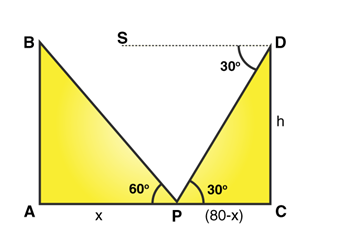Let AB and CD be the two poles of equal height standing on the two sides of the road of width 80 m.

AC = 80 m

Let AP = x then PC = 80 – x

P is a point on the road from which the angle of elevation of the top of tower AD is 60°, also, the angle of depression of the point P from the point B is 30°.

∠BPA = 60 o and ∠DPC = 30o

Draw a parallel line from DS to CA.

∠SDP = ∠DPC = 30°.

To Find : The height of the poles and the distance of the point P from both the poles.

From right △PAB:

tan 60 o = AB/AP

√3 = h/x

or h = x√3 …(1)

From right △PCD:

tan 30o = AB/AP

1/√3 = h/(80-x)

or h = (80-x)/√3 …(2)

Form (1) and (2), we have

(80-x)/√3 = x√3

80 – x = 3x

x = 20

Form (1): h = (20)√3

Therefore,

Height of each pole = (20)√3 m

Distance of pole AB from point P = 20 m

Distance of pole CD from point P = 80 – 20 = 60 m

Question 12: Two men are on opposite sides of a tower. The measure the angles of elevation of the top of the tower as 30° and 45° respectively. If the height of the tower is 50 metres, find the distance between the two men. [Take √3 = 1.732]

Solution:

Draw a figure based on given instructions: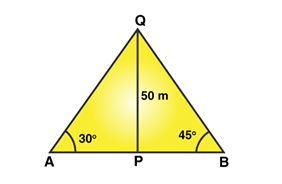Let the positions of the two men are A and B. Let PQ represent the tower.

∠QAP = 30° and ∠QBP = 45°.

Given: QP = 50 m.

We get two right-angled triangles ∆QPB and ∆QPA.

Now,

From right △QPA:

tan 300 = PQ/AP

1/√3 = 50/AP

AP = 50√3 = 86.6

From right ∆QPB:

tan450 = PQ/PB

1 = 50/PB

PB = 50

Now, AB = AP + PB

= 86.6 + 50

= 136.6

Therefore, the distance between the two men is 136.6 meters.

Question 13: From the top of a tower 100 m high, a man observes two cars on the opposite sides of the tower with angles of depression 30° and 45°, respectively. Find the distance between the cars. [Take √3 = 1.73]

Solution: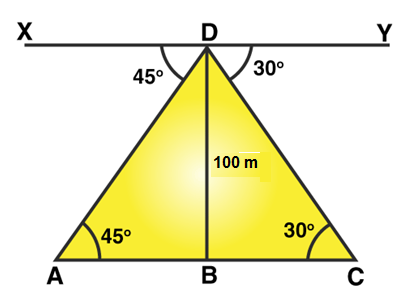In the figure,

A and C are the positions of the cars.

BD = 100 m.

The angles of depression are ∠DAB and ∠DCB.

Since the line XY is parallel to AC, we have

∠YDC = ∠DCB = 30° and

∠XDA = ∠DAB = 45°

To find: Distance between the two cars

From right ∆ABD:

tan450 = BD/AB

1 = 100/AB

or AB = 100

From right ∆CBD:

tan 300 = BD/BC

1/√3 = 100/BC

or BC = 100√3

Now, AC = AB + BC

= 100 + 100√3

= 100(1 + √3)

= 273.2

Hence distance between the cars is 273.2 meters.

Question 14: A straight highway leads to the foot of a tower. A man standing on the top of the tower observes a car at an angle of depression of 30°, which is approaching the foot of the tower with a uniform speed. Six seconds later, the angle of depression of the car is found to be 60°. Find the time taken by the car to reach the foot of the tower form this point.

Solution:

Draw a figure based on given instructions: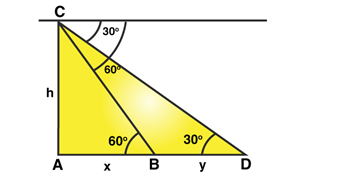In the figure, AC be the tower and AB be the canal.

Let AC = h, AB = x and BD = y

From right △CBA:

cot 60o = x/h

1/√3 = x/h

or x = h/√3

cot 30 o = (x+y)/h

√3 = (x+y)/h

y = 2h/√3

Since y distance is covered in 6 seconds, then x distance is covered in 6x/y seconds

Substituting the values of x and y, we get

6x/y = (6h/√3)/ (2h/√3)

= 3

Therefore, time taken by the car to reach the foot of the tower form this point is 3 seconds.

Question 15: A TV tower stands vertically on a bank of canal. From a point on other bank directly opposite the tower, the angle of elevation of the top of the tower is 60°. From another point 20 m away from this point on the line joining this point to the foot of the tower, the angle of elevation of the top of the tower is 30°. Find the height of the tower and the width of the canal.

Solution: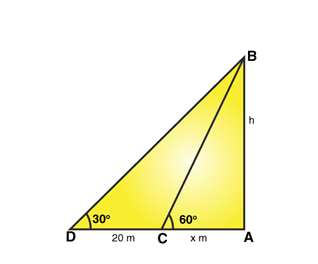In the figure, let AB be the tower and AC be the canal. C is the point on the other side of the canal directly opposite the tower.

Let AB = h, CA = x and CD = 20

From right △BAC:

cot 60o = x/h

1/√3 = x/h

x = h/√3 …(1)

√3 = (x+20)/h

x = h√3 – 20 …(2)

From (1) and (2), we have

h/√3 = h√3 – 20

h = 10√3

From (1): x = (10√3)/√3 = 10

Therefore, the width of the canal is 10 m and height of the canal is 10√3 m.

## R S Aggarwal Solutions for Chapter 14 Height and Distance Topics

In this chapter students will study important concepts on Height and Distance as listed below:

• Height and Distance introduction
• Angle of Elevation
• Angle of Depression
• Height and Distance word problems

### Key Features of R S Aggarwal Solutions for Class 10 Maths Chapter 14 Height and Distance

1. Solutions to chapter 14 have been solved as per the CBSE blueprint adhering to the textbook.

2. Diagrams are provided to promote visual learning.

3. All the questions are solved by subject experts.

4. These solutions will be useful for various competitive exams.